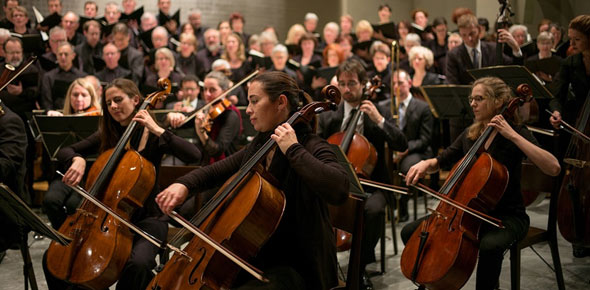# Musical Time Periods

20 Questions | Total Attempts: 27Settings.

Related Topics
• 1.
Which musical period occured between 1750 and 1820 A.D.?
• A.

A

• B.

B

• C.

C

• 2.
Which musical time period occured between 1600 and 1750 A.D.?
• A.

A

• B.

B

• C.

C

• 3.
1. Which musical era occured before A.D. 400?
• A.

A

• B.

B

• C.

C

• 4.
1. Which musical era occured before A.D. 400?
• A.

A

• B.

B

• C.

C

• 5.
1. Which musical era occured before A.D. 400?
• A.

A

• B.

B

• C.

C

• 6.
1. Which musical era occured before A.D. 400?
• A.

A

• B.

B

• C.

C

• 7.
1. Which musical era occured before A.D. 400?
• A.

A

• B.

B

• C.

C

• 8.
1. Which musical era occured before A.D. 400?
• A.

A

• B.

B

• C.

C

• 9.
1. Which musical era occured before A.D. 400?
• A.

A

• B.

B

• C.

C

• 10.
1. Which musical era occured before A.D. 400?
• A.

A

• B.

B

• C.

C

• 11.
1. Which musical era occured before A.D. 400?
• A.

A

• B.

B

• C.

C

• 12.
1. Which musical era occured before A.D. 400?
• A.

A

• B.

B

• C.

C

• 13.
1. Which musical era occured before A.D. 400?
• A.

A

• B.

B

• C.

C

• 14.
1. Which musical era occured before A.D. 400?
• A.

A

• B.

B

• C.

C

• 15.
1. Which musical era occured before A.D. 400?
• A.

A

• B.

B

• C.

C

• 16.
1. Which musical era occured before A.D. 400?
• A.

A

• B.

B

• C.

C

• 17.
1. Which musical era occured before A.D. 400?
• A.

A

• B.

B

• C.

C

• 18.
1. Which musical era occured before A.D. 400?
• A.

A

• B.

B

• C.

C

• 19.
1. Which musical era occured before A.D. 400?
• A.

A

• B.

B

• C.

C

• 20.
1. Which musical era occured before A.D. 400?
• A.

A

• B.

B

• C.

C